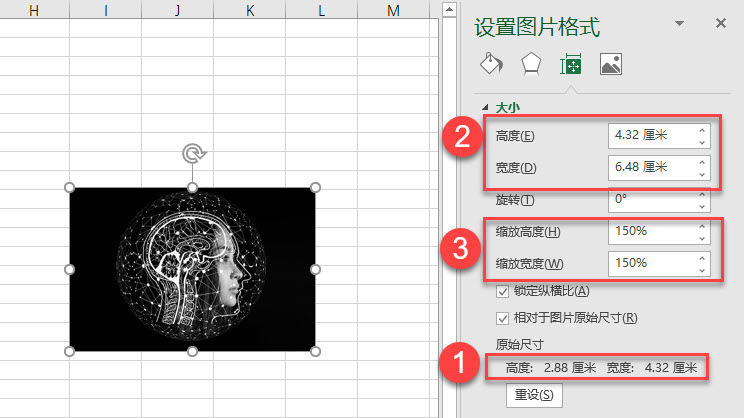﻿ 如何用vba获取图片的原始尺寸？ - EXCEL VBA - ExcelOffice【微信公众号：水星Excel】

# 如何用vba获取图片的原始尺寸？```Sub QQ1722187970()
Dim oSP As Shape
Dim oWK As Worksheet
Set oWK = Excel.ActiveSheet
Set oSP = oWK.Shapes(1)
With oSP
'之前的高和宽
iH = .Height
iW = .Width
.ScaleHeight 1, msoCTrue
.ScaleWidth 1, msoCTrue
'原来的高和宽
iHO = .Height
iWO = .Width
'输出原始的图片的高和宽，以厘米为单位
Debug.Print Point2Centimeters(iHO), Point2Centimeters(iWO)
'重置为图片一开始的尺寸
.Height = iH
.Width = iW
End With
End Sub
Function Point2Inch(ByVal dPoint As Double)
'Point转Inch
Point2Inch = dPoint * 1 / 72
End Function
Function Inch2Point(ByVal dInch As Double)
'Inch转Point
Inch2Point = dInch * 72
End Function
Function Point2Centimeters(ByVal dPoint As Double)
'Point转Centimeter
Point2Centimeters = dPoint * 1 / 72 * 2.54
End Function
Function Centimeters2Point(ByVal dCentimeter As Double)
'Centimeter转Point
Centimeters2Point = dCentimeter * 72 * 1 / 2.54
End Function
```